Free math worksheets of Simple Equations(सरल समीकरण) suitable for Grade 7 and 8.

An equation is a statement of equality between two mathematical expressions containing one or more variables. Simple Equations Class 6,7,8 Maths NCERT Solutions were prepared according to CBSE (NCERT) guidelines. Maths Class 7 Simple Equations

Free printable Simple Equations practice worksheets for class 6,7 & 8.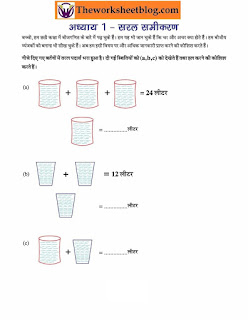free printable cbse maths worksheets.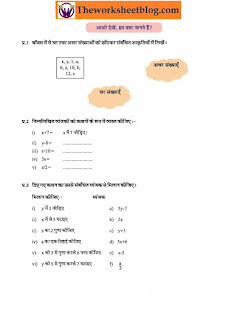simple equations worksheets for Hindi medium.saral samikaran practice worksheets.CBSE Class 7 ,8 Mathematics worksheets in hindi.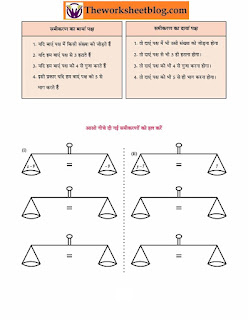CBSE Class 7 ,8 Mathematics worksheets in hindi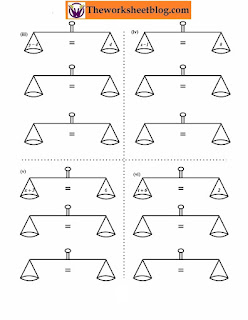CBSE Class 7 ,8 Mathematics worksheets in hindi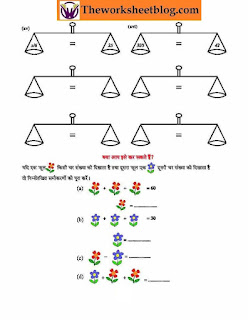simple equations practice worksheets.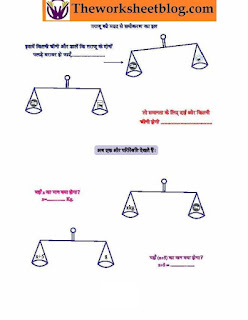saral samikaran practice worksheets.saral samikaran practice worksheets.saral samikaran practice worksheets. download more worksheets.